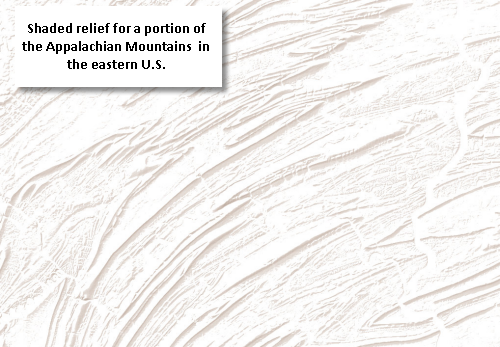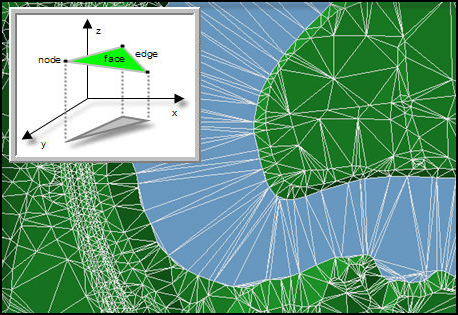Example: Representing surfaces
Example: Representing surfaces

This example illustrates how you might model surface elevation in a GIS. This is a useful example because you'll typically need to use multiple datasets for representing and analyzing surface elevation. Most of these are derived from a small set of data sources.

Typically, you will need the following:

• A digital elevation model (DEM) dataset stored as a raster. DEMs are used in surface analysis applications and for deriving other datasets for surface display.
• A triangulated irregular network (TIN). TINs are derived from data sources such as lidar and other 3D points, as well as 3D lines (such as water body shorelines, ridgelines, and so on).
• Contour lines and elevation points. These feature classes are derived from your DEM and are used for displaying surface elevation.
• A shaded relief raster that is derived from your DEM and used in your surface elevation display as well.

Continuous surfaces in GIS

A continuous surface describes an occurrence that has a value for every point on the earth. For example, surface elevation is a continuous layer of values for ground elevation above mean sea level for the entire extent of a dataset. Other surface type examples include rainfall, pollution concentration, population density, and subsurface representations of geologic formations.

Surface representation is somewhat challenging. With continuous datasets, it is impossible to represent all values for all locations. Various alternatives exist for representing surfaces using both features and rasters.

Often, a GIS will need to use multiple surface representations and datasets to depict surface elevation. For example:

Contour lines and elevation points—Contours are lines that represent locations having an equal value, such as surface elevation height. Elevation points can be used to represent key mountain peaks.Raster datasets—Each raster contains a matrix of cells where each cell value represents a measure of the continuous variable. For example, DEMs are frequently used to represent surface elevation and are often portrayed using shaded relief maps.TINs—A TIN is a data structure for representing surfaces as a connected network of triangles. Each triangle node has an x,y coordinate and a z or surface elevation value. TINs can contain additional information for modeling surface elevation such as ridgelines, drainage lines, building pads, and water boundaries. Like a DEM, TIN representations can be used to estimate the surface value for any location using interpolation.Source: http://help.arcgis.com